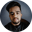Related Tags

golang
go
random
communitycreator

# How to generate random numbers in a given range in GoShubham Singh Kshatriya

### Overview

Go provides a built-in package named math/rand to generate pseudorandom numbers.

The pseudorandom numbers are a deterministic sequence of numbers that depend on a seed value. In order to generate random numbers on every execution, we need to set the seed every time.

In this shot, we’ll see how to generate a random number in a given range using the rand.Intn() function of the math/rand package.

### rand.Intn() function

rand.Intn(n)


The rand.Intn() function accepts a number n and returns an unsigned pseudorandom integer in the interval [0, n). It will throw an error if the value of n is less than zero.

### Generate random number in a specific range

We can use the following syntax to generate a random number in a specific range.

rand.Intn(max-min) + min


Here, max is the upper bound and min is the lower bound. It will return a random number in the interval [min, max).

### Code

Let’s see an example to generate a random number between 1 and 5.

#### Packages

We will use the following packages.

• fmt formats the output.
• math/rand generates random numbers.
• time sets the seed.
package main

import (
"fmt"
"math/rand"
"time"
)

func main() {
min := 1
max := 5
// set seed
rand.Seed(time.Now().UnixNano())
// generate random number and print on console
fmt.Println(rand.Intn(max - min) + min)
}

### Explanation

• Lines 3–7: We import all the necessary packages.
• Lines 10–11: We declare 2 variables min and max that specify the lower and the upper bound respectively.
• Line 13: We set a seed using time.Now().UnixNano() function. This function will return the number of seconds passed from January 1, 1970.
• Line 15: We generate the random number using the rand.Intn() function and output it on the console.

RELATED TAGS

golang
go
random
communitycreator

CONTRIBUTORShubham Singh Kshatriya
RELATED COURSES

View all Courses

Keep Exploring

Learn in-demand tech skills in half the time